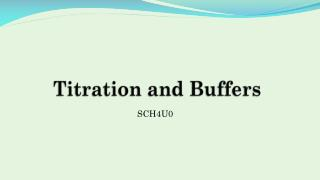DownloadDownload PresentationTitration and Buffers

# Titration and Buffers

Télécharger la présentation## Titration and Buffers

- - - - - - - - - - - - - - - - - - - - - - - - - - - E N D - - - - - - - - - - - - - - - - - - - - - - - - - - -
##### Presentation Transcript

1. Titration and Buffers SCH4U0

2. Titration • Titration is an experimental method of determining the concentration of an unknown solution. • It requires two compounds that will react with one another • You must know the amounts added of both and the concentration of one solution • It can be used with acids and bases since they react with one another • We should be able to calculate the pH of the solution at any point in the titration

3. Strong Acid with Strong Base • When a strong acid is titrated with a strong base, a graph of pH versus volume of base added looks like this: • The most important part of this graph is the inflection point • This is called the equivalence point • The equivalence point is the point where exactly enough base has been added to react all the base (no excess)

4. Strong Acid with Strong Base • Notice that the equivalence point is at pH 7 • This occurs only if a strong acid is titrated with a strong base • There are no acids/bases at equivalence • So pH will be 7

5. Solving pH – Strong/Strong • What is the pH when 1.00 mL of 0.10 M is added to 10.00 mL of 0.10 M ? • Use stoichiometry to determine what is left over after the reaction

6. Solving pH – Strong/Strong • To solve for pH we need the hydronium concentration • Therefore, at this point in the titration, the pH will be 1.09

7. Weak Acid with Strong Base • When a weak acid is titrated with a strong base, a graph of pH versus volume of base added looks like this: • Notice that the equivalence point is above seven • There is a base at the equivalence point • Also, there is a sharp rise in pH at the beginning and then it rise slowly

8. Solving pH – Weak Acid • What is the pH when 1.00 mL of 0.10 M is added to 10.00 mL of 0.10 M ? • Use stoichiometry to determine what is left over after the reaction

9. Solving pH – Weak Acid • Now that the hydroxide is used up, what will happen? • We have a weak acid that will dissociate in water • We can use this to determine pH

10. Sample Problem

11. Solving pH – Weak Acid • What about at equivalence? What is the pH? • We would need 10.00 mL of 0.10 M to be added to 10.00 mL of 0.10 M . • Use stoichiometry to determine what is left over after the reaction

12. Solving pH – Weak Acid • Now that the hydroxide is used up, what will happen? • We only have a weak base () that will dissociate in water • We can use this to determine pH

13. Solving pH – Weak Acid The assumption works

14. Weak Base with Strong Acid • When a weak base is titrated with a strong acid, a graph of pH versus volume of acid added looks like this: • Notice that the equivalence point is below seven • There is an acid at the equivalence point • Also, there is a sharp drop in pH at the beginning and then it rise slowly

15. Buffers • When weak acids/bases are titrated there is a large region where the pH barely changes before the equivalence point • This is called the buffer zone • Because a buffer is generated • A buffer is a solution that resist pH changes • It does this because it contains a weak acid and a weak base • They are often conjugates of each other, but amphoteric compounds can be used too

16. Buffers • Example: • A solution that contains 0.50 M and 0.50 M would be a buffer. • If an acid were added to the buffer, it would react with . Once they acid were consumed it would not be able to dissociate to produce hydronium • Ie: pH would not change (much) • If a base were added to the buffer, it would react with . There would be no generation of hydroxide • And little to no pH change